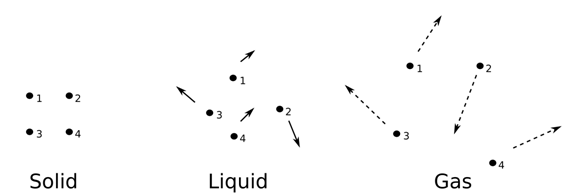Behavior of gas molecules and ideal gas equation

We have read about the physical properties of matter in our previous classes that included their flowability, their shape and density. As we know, the molecules of gas are loosely packed, having the least density and the greatest inter molecular distance as compared to solids and liquids.Because of this, the mutual interaction between two molecules of a gas is negligible and at low pressure and temperature, they satisfy the relation between pressure, temperature and volume as given by the following equation.

$PV=KT$

Where P is the pressure of the gas, V is the volume of the gas, T is the temperature at which the system is kept, measured in Kelvin and K is the proportionality constant which depends on the nature of gases contained in the system. Further, K is proportional to the number of molecules in the sample, so we can write this mathematically as,

$\frac{PV}{KT}=constant=k_{b}$

Where N is the number of molecules in the sample and k is another constant of proportionality. The studies performed on k suggest that the value of k remains the same for all the gasses and is defined as the Boltzmann constant denoted as kb.

Here we can see that,

This implies that, if P, V and T are the same, the number of molecules in the system N is the same. This proves the Avogadro’s hypothesis that for a unit volume of gasses at a fixed temperature and pressure, the number of molecules per unit volume of the gas is the same. For 22.4 liters of gas at standard temperature and pressure, this number is equal to 6.023 × 1023, which is known as the Avogadro’s number. This amount of a substance is termed as the 1 mole of that substance i.e., 6.023 × 1023 atoms of oxygen are termed as 1 mole of the oxygen atom.

This has been further proven by the kinetic theory of gasses. As per the gas equation suggested by the kinetic theory of gasses, PV=nRT , where n is the number of moles of gasses, R is the universal constant with a value equal to 8.314 Jmol-1K-1 and T is the temperature in Kelvin.